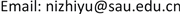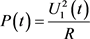Vibration Suppression and Energy Harvesting of Large Flexible Spacecraft under Complex Environments

Beichao Wang, Yuhang Zhang, Zhiyu Ni, Bo Fang

College of Aerospace Engineering, Shenyang Aerospace University, Shenyang LiaoningReceived: Jan. 6th, 2021; accepted: Feb. 25th, 2021; published: Mar. 3rd, 2021ABSTRACT

This study proposed Nonlinear Energy Sink (NES) for the vibration suppression of large flexible spacecraft in complex environments, and Giant Magnetostrictive Materials (GMM) for harvesting the vibrational energy dissipated by NES to reach the effect of energy saving. Considering the influence of sunlight pressure and thermal shock, the in-orbit dynamic model of large flexible spacecraft was established in the Hamilton system. The NES equations of vibration suppression were obtained according to Newton’s dynamic laws, and introducing Jiles-Atherton model deduced the GMM expressions of energy harvesting. NES-GMMs were coupled to the both free ends of spacecraft, which was discretized by Galerkin method, and Runge-Kutta method was used for numerical simulations. The results demonstrated that the effect of NES on the vibration suppression of spacecraft is greatly significant. Meanwhile, GMM can harvest parts of the energy dissipated by NES. Therefore, the proposed method is completely feasible. Moreover, properly adjusting the structural parameters of NES and GMM can also improve the working performance. This study provides a new idea for the application of the technologies of vibration control and energy harvesting in the aerospace field.

Keywords:Large Flexible Spacecraft, Nonlinear Energy Sink, Vibration Suppression, Giant Magnetostrictive Energy Harvesting1. 引言

2. 大型挠性航天器在轨动力学建模

(1) 在本文中，地球被视为一个理想球体。

(2) 航天器以特定的轨道绕地球运行。

(3) 航天器的长度远大于厚度。

(4) 忽略航天器的扭转和剪切变形。

r P = A e o R 1 + A e o A o a ( ρ P + w P ) (1)

A e o = [ cos θ 0 sin θ 0 1 0 − sin θ 0 cos θ ] , A o a = [ 1 0 0 0 cos φ − sin φ 0 sin φ cos φ ] (2)

T P = 1 2 m P d r P T d t ⋅ d r P d t (3)

T = 1 2 ∫ { ( ∂ w ∂ t ) 2 + ( ω 0 R 1 ) 2 + ( w 2 + x 2 ) ( ω 0 + d φ d t ) 2 + 2 ω 0 R 1 ∂ w ∂ t cos φ + [ 2 ω 0 R 1 ( x cos φ − w sin φ ) + 2 x ∂ w ∂ t ] ( ω 0 + d φ d t ) } d m 1 (4)

U d = 1 2 ∫ E I ( ∂ 2 w ∂ x 2 ) 2 d x , U g = − ∫ μ r d m 1 (5)

r = R 1 2 + x 2 + w 2 + 2 x R 1 cos φ − 2 w R 1 sin φ (6)

F s = ∮ A d f s , T s = ∮ A r s × d f s (7)

d f s = ( 2 3 C r d + 2 C r s cos β ) n ρ s d A + p 1 H ( cos β ) cos β ( a s + C r d ) r s (8)

W s = ∫ 0 π 2 T s d φ , β = 0 (9)

k t ∂ 2 T ∂ z 2 + δ q t = ρ c t ∂ T ∂ t (10)

Q = c t m 1 Δ T = α t S H t t (11)

W t = ∫ 0 L ∫ 0 h E α t α e S H t c t m 1 y d y ∂ y ∂ x d x (12)

∫ t 1 t 2 ( δ T − δ U + δ W ) d t = 0 (13)

∫ [ d 2 φ d t 2 ( x 2 + w 2 ) + 2 w ( ω 0 + d φ d t ) ∂ w ∂ t + ( W s + W t ) + ( ω 0 2 − μ r 3 ) R 1 ( x sin φ + w cos φ ) + x ∂ 2 w ∂ t 2 ] d x = 0 (14)

∂ 2 w ∂ t 2 + [ μ r 3 − ( ω 0 + d φ d t ) 2 ] w + x d 2 φ d t 2 + ( W s + W t ) + E I ρ A ⋅ ∂ 4 w ∂ t 4 + ( ω 0 2 − μ r 3 ) R 1 sin φ = 0 (15)

3. NES振动抑制与GMM能量采集

m z ¨ + c ( z ˙ − w ˙ ) + k ( z − w ) 3 = 0 (16)

F N E S = c ( w ˙ − z ˙ ) + k ( w − z ) 3 , W N E S = ∫ 0 L F N E S w d x (17)

E l i n = w ˙ 2 2 + ε z ˙ 2 2 + w 2 2 + k L ( w − z ) 2 2 (18)

E N E S = w ˙ 2 2 + ε z ˙ 2 2 + w 2 2 + ε k ( w − z ) 4 4 (19),(31)

4. 数值仿真

Structural and spatial parameters of large flexible spacecraft [<xref ref-type="bibr" rid="hanspub.40782-ref21">21</xref>] [22

Structural parameters of NES-GMM [<xref ref-type="bibr" rid="hanspub.40782-ref20">20</xref>] [23

GMM棒长度0.5 m
GMM棒直径0.2 m
GMM棒弹性模量5 × 1010 Pa

5. 结论

(1) 利用NES对大型挠性航天器进行振动抑制是非常可行的。提高NES的阻尼比会显著增强自身的抑制性能，最快可在20 s内完成航天器的振动抑制，相比之下NES的非线性刚度对其抑制性能无明显影响，结果体现了NES高效、稳定的特性。

(2) GMM能量采集技术用于大型挠性航天器同样是可行的。GMM具有良好的采集性能，其性能与磁致伸缩系数和磁导率均呈正相关，经对比，磁致伸缩系数对于GMM性能的提升更显著，优化后GMM采集的振动能总量占NES耗散的77%以上，采集时间可延长至75 s。

(1) 大型挠性航天器的一个重要特点是模态密集，由于结构复杂，模型的准确性也难以保证，对多个模态进行控制时独立模态空间的控制效果可能不佳，而对整个系统进行控制器设计的难度也较大。因此需要进一步结合分布式控制方法，进一步研究结构振动控制方法。

(2) 由于大型挠性航天器的控制性能指标大大提高，系统对控制精度和稳定度的要求也逐渐提高，这些系统特性对大型航天器的控制系统设计提出了严峻挑战。因此需要基于航天器的振动控制，在大挠性结构中加入嵌入式作动器，进一步研究系统布局优化、执行机构配置与布局优化等问题。